## 4.6.2 Scattering with Acoustic Phonons

Interaction with acoustic phonons (AP) can be approximated as elastic scattering,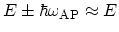. As discussed in Section 2.6, near the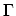-point a linear dispersion relation for acoustic phonons is assumed,, where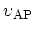is the acoustic phonon velocity. Furthermore, at room temperature low energy phonons have an appreciable occupation, such that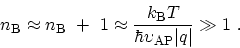(4.45)

With equation (4.45) and the elastic approximation the contributions due to phonon emission and absorption become equal and can be lumped into one term. As a result, by using the relations (2.15) and (2.19) the self-energies due to acoustic phonon interaction are written as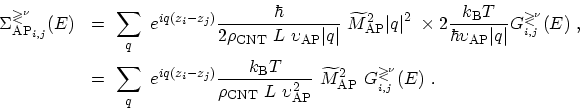(4.46)

With the exception of the exponential term all terms in (4.46) can be taken out of the sum and one can convert the sum into an integral over q, see (4.38) and (4.39). The self-energies simplify to(4.47)

where similar to (4.42)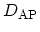is given by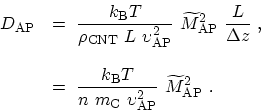(4.48)

A discussion similar to that in Section 4.6.1 gives a justification to keep only diagonal elements of the self-energy due to the interaction of electrons with acoustic phonons.

By substituting (4.47) in (3.76) the retarded self-energy is obtained as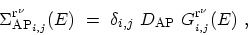(4.49)

Due to the approximations made the retarded self-energy for scattering with acoustic phonons is simplified and directly related to the retarded GREEN's function.Therefore, one does not need to evaluate the integrals like (4.43), which implies a considerable saving of computational cost. M. Pourfath: Numerical Study of Quantum Transport in Carbon Nanotube-Based Transistors